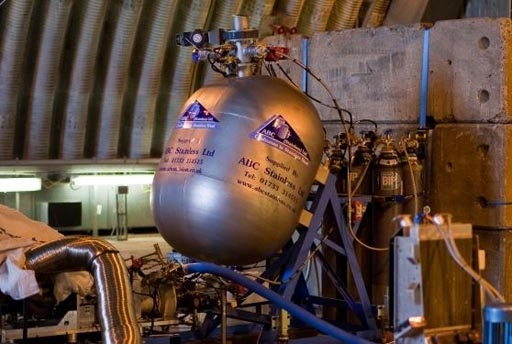# Fuel Tank

Maths Question of the WeekWednesday, 28 January, 2015

The engineers at BLOODHOUND SSC mounted an experimental fuel tank on a test rig at an angle to simulate the effects of acceleration on a fuel load. The tank is partially filled with fuel.The cross section of the tank shown below has a 100cm long base, is 60 cm wide and 40 cm high. They tilted it as shown, resting on its 60 cm edge, with the fuel level reaching the midpoint of the base.

If they rested the tank down to a horizontal position, what would the depth of the fuel be in cm?

(you can assume for this question that the tank is a rectangular cuboid)

Answer

The volume of the tank is calculated as Base X Height X Width

100 X 40 X 60 = 240,000 cm3

In our example, the volume of the fuel rises half way up the inclined base. That is 50cm along the base.

It angles diagonally to the top left hand corner of the angled height halving this volume.

We therefore have to find half the volume of this rectangle:

50 cm X 40 cm X 60 cm = 120,000 cm3

To find this calculate; ½ base X height X length

½ X 50 X 40 X 60 = 60,000 cm3

60,000 cm3 is one quarter of the total volume of the tank of 240,000 cm3

If we lay the tank horizontally then we will see that the volume of the fuel will rise one quarter of the height of the tank of 40 cm.

Therefore the depth of the fuel is a quarter of 40 cm which is 10 cm